# MO8-Z8-I-5 2017

Identical rectangles ABCD and EFGH are positioned such that their sides are parallel to the same. The points I, J, K, L, M and N are the intersections of the extended sides, as shown. The area of the BNHM rectangle is 12 cm2, the rectangle MBCK area is 63 cm2 and the rectangle MLGH area is 28 cm2. Find the area of the IFJD rectangle.

Correct result:

S =  418 cm2

#### Solution: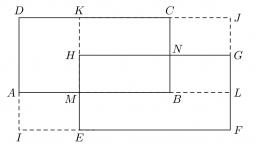We would be very happy if you find an error in the example, spelling mistakes, or inaccuracies, and please send it to us. We thank you!#### You need to know the following knowledge to solve this word math problem:

We encourage you to watch this tutorial video on this math problem:

## Next similar math problems:

• Irregular pentagon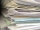A rectangle-shaped, 16 x 4 cm strip of paper is folded lengthwise so that the lower right corner is applied to the upper left corner. What area does the pentagon have?
• Gardens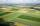I have a garden in the shape of a square with a side length of 0.02 kilometers. Neighbor has a large garden as well and one side of the garden we share. I'd bought from a neighbor garden that my area of my garden increased to 5 ares. a/ By how many acres
• Window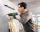Peter demolish box hole in the wall for window frame 147×51 cm. When they came masters fitted frame and window will be found that glass is 13.3 cm from the hole in each direction. Calculate how much percent less light enters the window than hole?
• Tiles path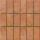A rectangular park measures 24mX18m. A path 2.4m wide is constructed all around it. Find the cost of paving the path with cement tiles of size 60cm X 40cm at the rate of \$5.60 per tile ?
• Square to rectangle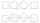What is the ratio of the area of a square of side x to the area of a rectangle of a rectangle of width 2 x and length 3
• Rectangle vs square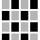The rectangle has dimensions of 13 × 10, square 8 × 8. Which shape has more area and how much above?
• Rectangular fieldOne dimension of the rectangular field is 56 m greater than second dimension. If each side of the rectangle increases by 10 m, increases the surface field is 1480 m2 . Determine dimencions of the field.
• Rectangular land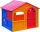On a rectangular land with dimensions of 35 m and 18.5 m is a house with a square floor plan with a side of 14 m. What % of the land is not occupied?
• Playground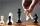On the special playground, there are 81 square sectors, each with a side of 5 m. How many players can fit on the playground if each player needs a 75 m2 area to play?
• Sidewalk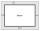In the garden, a 1-meter-wide sidewalk will be paved with tiles around the block-shaped pool. In the picture, the sidewalk is shown in gray. The dimensions of the bottom of the pool are 8.5 meters and 6 meters. The height of the pool walls is 2 meters. Ho
• Playground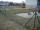Fencing square playground cost € 464; 1 meter cost € 19. What is the area of the playground?
• ScreensThe area of a 25 inch TV screen is 300 inch² the area of a 40 inch TV screen is 768 inch². The area of the smaller screen is what percent of the area of the larger screen?
• Two 2D shapes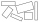Decide which shapes have more area:   (a) a square of 8cm side; or   (b) two rectangles with sides 5cm and 15cm? Write result as 1 or 2 (rectangles)
• Two rectangles 2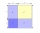A square of area 36 cm2 is cut out to make two rectangles. A and B The area of area A to area B is 2 : 1 Find the dimensions of rectangles A and B.
• Edge of prismThe regular quadrilateral prism has a surface of 250 dm2, its shell has a content of 200 dm2. Calculate its leading edge.
• Tunnels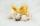Mice had built an underground house consisting of chambers and tunnels: • each tunnel leading from the chamber to the chamber (none is blind) • from each chamber lead just three tunnels into three distinct chambers, • from each chamber mice can get to any
• MO 2016 Numerical axisCat's school use a special numerical axis. The distance between the numbers 1 and 2 is 1 cm, the distance between the numbers 2 and 3 is 3 cm, between the numbers 3 and 4 is 5 cm and so on, the distance between the next pair of natural numbers is always i